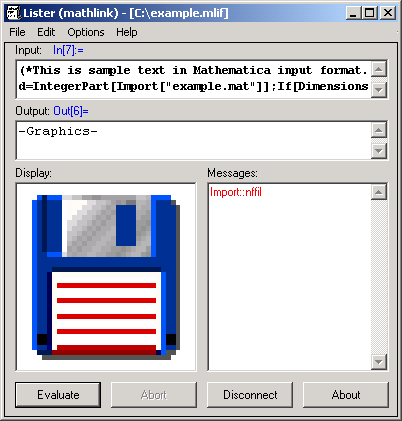The Mathematica® Link plugin acts like a simple Mathematica front end. It is able to load and evaluate files which are either in Mathematica input format (InputForm, .mlif) or in Mathematica binary format (DumpSave, .mx).

Evaluation requires a running connection to the Mathematica kernel provided by the MathLink® interface.